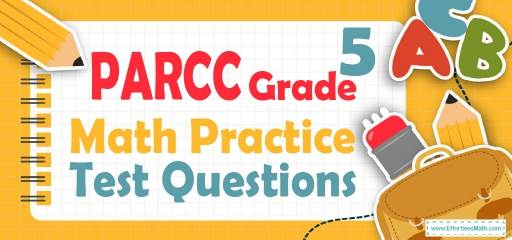# 5th Grade PARCC Math Practice Test QuestionsPreparing your student for the 5th Grade PARCC Math test? Help your students build PARCC Math test skills by following common 5th Grade PARCC Math questions. Practicing common math questions is the best way to help your students improve their Math skills and prepare for the test. Here, we provide a step-by-step guide to solve 10 common PARCC Math practice problems covering the most important math concepts on the 5th Grade PARCC Math test.

## 10 Sample 5th Grade PARCC Math Practice Questions

1- What is the volume of this box?

A. $$24 \space cm^3$$

B. $$32 \space cm^3$$

C. $$162 \space cm^3$$

D. $$192\space cm^3$$

2- How many square feet of tile is needed for a 18 feet to 18 feet room?

A. 72 Square Feet

B. 108 Square Feet

C. 216 Square Feet

D. 324 Square Feet

3- Of the 2,400 videos available for rent at a certain video store, 600 are comedies. What percent of the videos are comedies?

A. $$18 ½ \%$$

B. $$20 \%$$

C. $$22 \%$$

D. $$25 \%$$

4- How many $$3 × 3$$ squares can fit inside a rectangle with a height of 54 and width of 12?

A. 72

B. 62

C. 50

D. 44

5- ABC Corporation earned only $200,000 during the previous year, two–third only of the management’s predicted income. How much earning did the management predict? A.$20,000

B. $30,000 C.$300,000

D. $340,000 6- William keeps track of the length of each fish that he catches. Following are the lengths in inches of the fish that he caught one day:$$13, 14, 9, 11, 9, 10, 18$$ What is the median fish length that William caught that day? A. 11 Inches B. 9 Inches C. 12 Inches D. 13 Inches 7- $$9 + [8 × 5] ÷ 2 =$$ ? __________ 8- What is the median of these numbers? $$4, 9, 13, 8, 15, 18, 5$$ A. 8 B. 9 C. 13 D. 15 9-The area of the base of the following cylinder is 50 square inches and its height is 10 inches. What is the volume of the cylinder? __________ 10- A rope 13 yards long is cut into 4 equal parts. Which expression does NOT equal to the length of each part? A. $$13 ÷ 4$$ B. $$\frac{13}{4}$$ C. $$4 ÷ 13$$ D. $$4\sqrt{13}$$ ## The Absolute Best Book to Ace the 5th GradePARCCMath Test ## Answers: 1- D Use volume of cube formula. $$Voluem= length × width × height ⇒ V= 6 × 4 × 8 ⇒ V=192 \space cm^3$$ 2- D Find the area of the room which is a square. Use area of square formula. $$S= a^2 ⇒ S= 18$$ feet $$× 18$$ feet $$= 324$$ square feet 3- D Use percent formula: $$part =\frac{percent}{100}× whole$$ $$600 =\frac{percent}{100}× 2400⇒ 600= percent × 24 ⇒percent=25$$ 4- A Use area of rectangle formula. $$A= a × b ⇒ A= 54 × 12 ⇒ A= 648$$ Divide the area by 9 ($$3 × 3 = 9$$ squares) to find the number of squares needed. $$648 ÷ 9 = 72$$ 5- C ABC Corporation’s income = $$\frac{2}{3}$$ management’s predicted income. $$200,000 = \frac{2}{3}$$ management’s predicted income management’s predicted income $$= 200,000×\frac{3}{2} =300,000$$ 6- A Write the numbers in order: $$9, 9, 10, 11, 13, 14, 18$$ Median is the number in the middle. Therefore, the median is 11. 7- 29 Use PEMDAS (order of operation): $$9 + [8 × 5] ÷ 2 = 9 + (40) ÷ 2 = 9 + (40 ÷ 2) = 29$$ 8- B Write the numbers in order: $$4, 5, 8, 9, 13, 15, 18$$ Median is the number in the middle. Therefore, the median is 9. 9- 500 Use volume of cylinder formula. $$Voluem= base × heigth ⇒ V=50 × 10 ⇒ V=500$$ 10- C 13 yards long rope is cut into 4 equal parts. Therefore, 13 should be divided by 4. Only option C is NOT 13 divided by 4. (It is 4 divided by 13) Looking for the best resource to help you succeed on the 5th Grade PARCC Math test? ### The Best Books to Ace the 5th GradePARCC Math Test ## Related to This Article ### More math articles ### What people say about "5th Grade PARCC Math Practice Test Questions - Effortless Math: We Help Students Learn to LOVE Mathematics"? No one replied yet. X 30% OFF Limited time only! Save Over 30% SAVE$5

It was $16.99 now it is$11.99